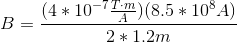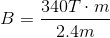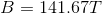# High School Physics : Understanding the Relationship with Current

## Example Questions

### Example Question #1 : Magnetism And Electromagnetism

A current of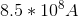runs through a straight wire. If the resulting magnetic field has a radius of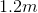, how strong is the magnetic field?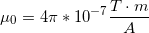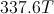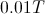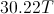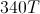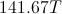Explanation:

Ampere's law states: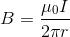.

In other words, the magnetic field (), is equal to a constant (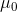) times the current (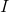) divided by the circumference of the magnetic field it is creating.

We are given the current, the constant, and the radius. Using these values, we can solve for the magnetic field strength.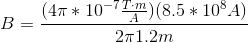Notice that thecancels out.